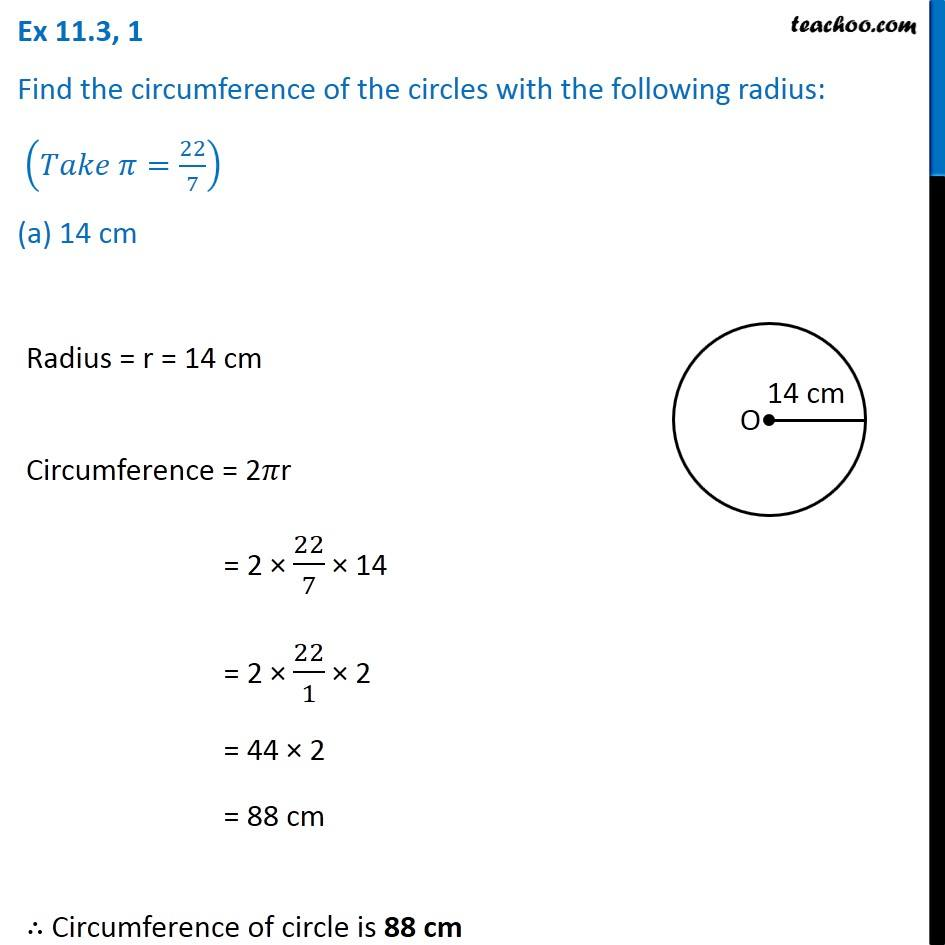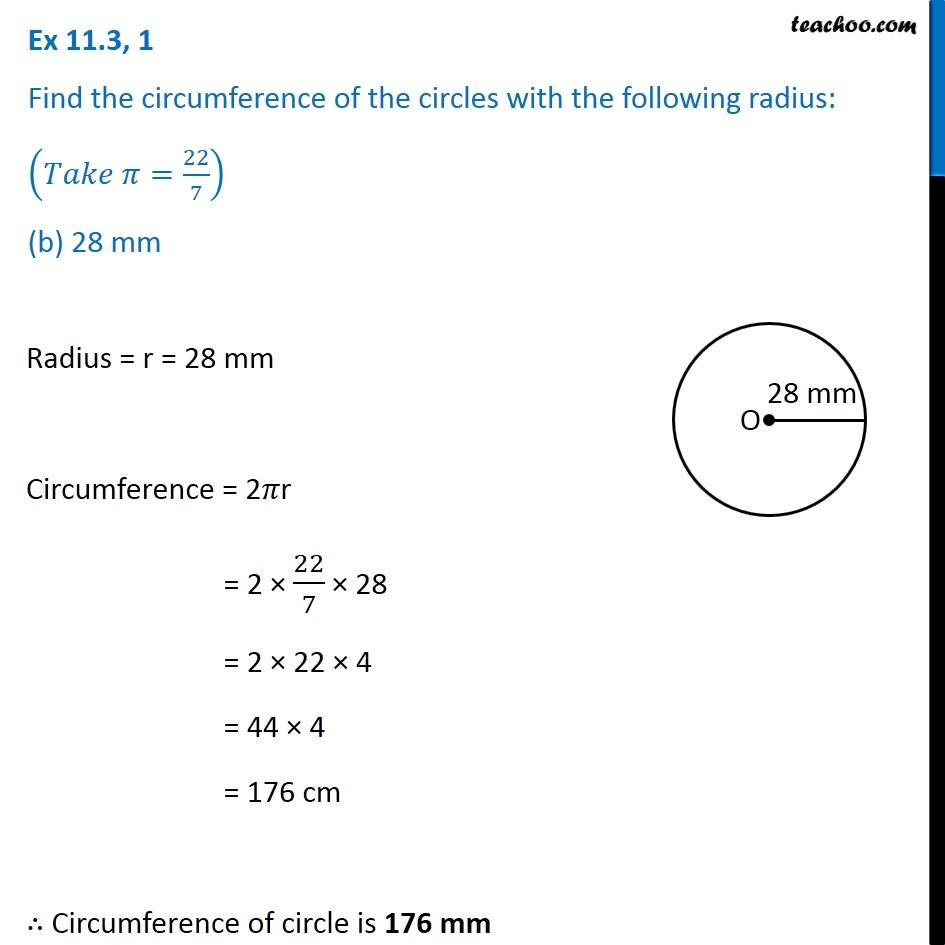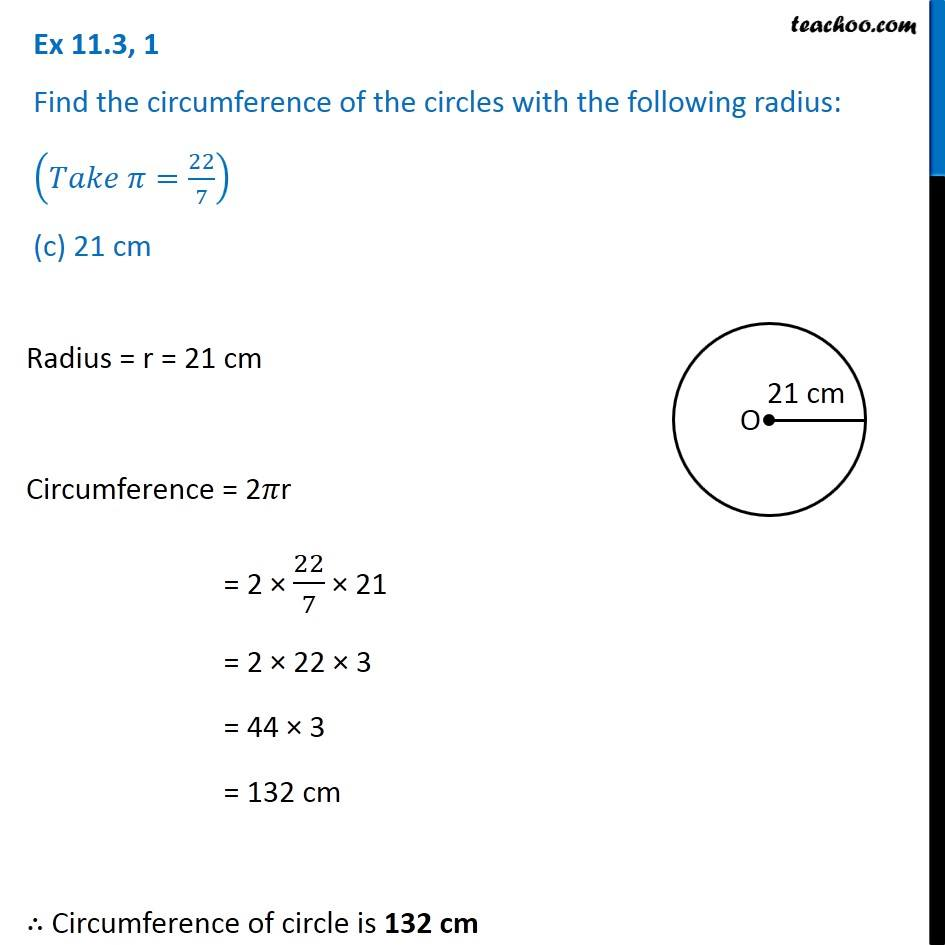Subscribe to our Youtube Channel - https://you.tube/teachoo

1. Chapter 11 Class 7 Perimeter and Area
2. Serial order wise
3. Ex 11.3

Transcript

Ex 11.3, 1 Find the circumference of the circles with the following radius: (𝑇𝑎𝑘𝑒 𝜋=22/7) (a) 14 cm Radius = r = 14 cm Circumference = 2𝜋r = 2 × 22/7 × 14 = 2 × 22/1 × 2 = 44 × 2 = 88 cm ∴ Circumference of circle is 88 cm Ex 11.3, 1 Find the circumference of the circles with the following radius: (𝑇𝑎𝑘𝑒 𝜋=22/7) (b) 28 mm Radius = r = 28 mm Circumference = 2𝜋r = 2 × 22/7 × 28 = 2 × 22 × 4 = 44 × 4 = 176 cm ∴ Circumference of circle is 176 mm Ex 11.3, 1 Find the circumference of the circles with the following radius: (𝑇𝑎𝑘𝑒 𝜋=22/7) (c) 21 cm Radius = r = 21 cm Circumference = 2𝜋r = 2 × 22/7 × 21 = 2 × 22 × 3 = 44 × 3 = 132 cm ∴ Circumference of circle is 132 cm

Ex 11.3